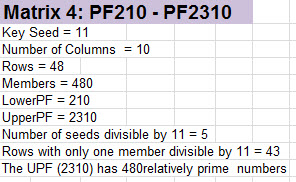Big Prime Number

# Matrix 4

It is only with Matrix 4 that we can see the importance of including as seeds the non-prime numbers from the prior matrix, the ones in pink blocks (ex. 121,143 etc.). These were the numbers not divisible by the Key Seed number but the ones that were also not prime. It turns out that these numbers are also valid seed numbers and when the lower PF is added to one of these numbers, it also generates either a prime or a not prime number relatively prime number to the upper PF. Although these numbers share equally in the computation of higher matrix members, they themselves could never be Key Seed numbers as they will be removed from the seed list immediately after their prime factor is used as the Key Seed in a matrix. So for example in Matrix 3 we see the numbers 49, 77, 91 etc.(all divisible by 7) removed from the seed list in Matrix 4. Rule 4 is that any number relatively prime to a PF(x) number is a seed number for the next higher matrix PF(x+1).

Based on what has been explained we can very easily derive sets of numbers that are likely to contain prime numbers. Using the knowledge that the numbers appear in pairs in a matrix to add up to the upper PF number, we can work backward for instance and start with the upper PF number and subtract seed numbers in order to locate the next possible prime numbers smaller than the upper PF number. In Matrix 4, we can subtract 1 from the upper PF 2310 to get 2309. Similarly subtracting the next seed number 11 from 2310 produces 2299. These are the 2 highest numbers smaller than the upper PF number that are relatively prime to 2310 and are candidates for being prime. Taking this case to an extreme example we could take the largest prime number ever found which is on the order of 11 million digits and begin subtracting seed numbers from the next higher PF number and we would find a prime number that would set the all-time record. However it gets even better because each of those numbers is directly related to the seed numbers that created them and can be traced back to those seeds by simply subtracting the next smaller PF number. So in this case using Matrix 4 as an example, with number 2299, we can subtract 210 numerous times and find 2089, 1879, 1669 etc. until we reach 199 which is the seed number. Although it is probably obvious, it bears pointing out that this seed number itself is exactly 210 minus 11. Each of the numbers that are computed in this example are themselves candidates for being prime. So aside from the potential enormous computational effort to prove that an 11 million digit number is actually prime, it is trivial to select the members of the matrix that guarantee finding the largest prime number of record since nearly every single one that would be examined is relatively prime to the upper PF number (except the very few that are divisible by the Key Prime). This can hold true for any size PF number, be it 4 digits or 400 million digits. There is absolutely no chance of wasting any computational effort in examining any number that is not a member of this exclusive club of numbers. That said, it is still true that there are likely to be members of the matrix that are not easily discovered if the matrix is so large that the seed

numbers are not all known, particularly if some of those numbers themselves are very large prime numbers. As the list of seed numbers grows in the larger matrices, it is clear that most of the seed numbers themselves are not prime numbers. Those non-prime seeds are relatively easy to compute. As an example in Matrix 4 we know that 13×13 = 169 and that is the seed for 4 of the larger prime numbers in its row. In very large matrices we would find that a large seed number might be the multiple of either many seed numbers or a smaller prime number raised to a large power.## Compute the Number of Matrix Members

The rules that I have established allow for a simple recursive formula to compute the exact number of members of a matrix. As I have shown, this is the same thing as the number of numbers relatively prime to a PF although they are different lists as one includes the seeds (but no numbers factored by the Key Seed) and the other does not. Below I provide the rules for this computation.

• Let X be the number of the Matrix
• Let M(X) be the number of members of matrix X
• Let UPF(X) be then upper PF of matrix X.
• Let RP(X) be the count of the number of numbers relatively prime to the Upper PF
• We know that RP(X) = M(X) (different lists but same count)
• The members of a matrix is the column count (excluding seed column) times the row count.
• Let R(X) = row count of matrix X
• Let C(X) = column count of matrix X
• M(X) = R(X) * C(X)
• Let KS(X) be the Key Seed (smallest prime) in the seed column of matrix X
• The number of columns in a matrix is 1 less than the smallest prime (Key Seed).
• C(X) = KS(X) – 1
• The number of rows in matrix X is equal to the number of members of matrix (X-1)
• R(X) = M(X-1)

• RP(X) = M(X)
• RP(X) = R(X)*C(X)
• RP(X) = M(X-1)*(KS(X)-1)

X KS(X) KS(X)-1 M(X)
1 3 2 2
2 5 4 8
3 7 6 48
4 11 10 480
5 13 12 5760

Just to reiterate, the simple recursive formula to compute members of each matrix is to multiply the number of members of the prior matrix by 1 less than the Key Seed of that matrix.

RP(X) = M(X-1)*(KS(X)-1)